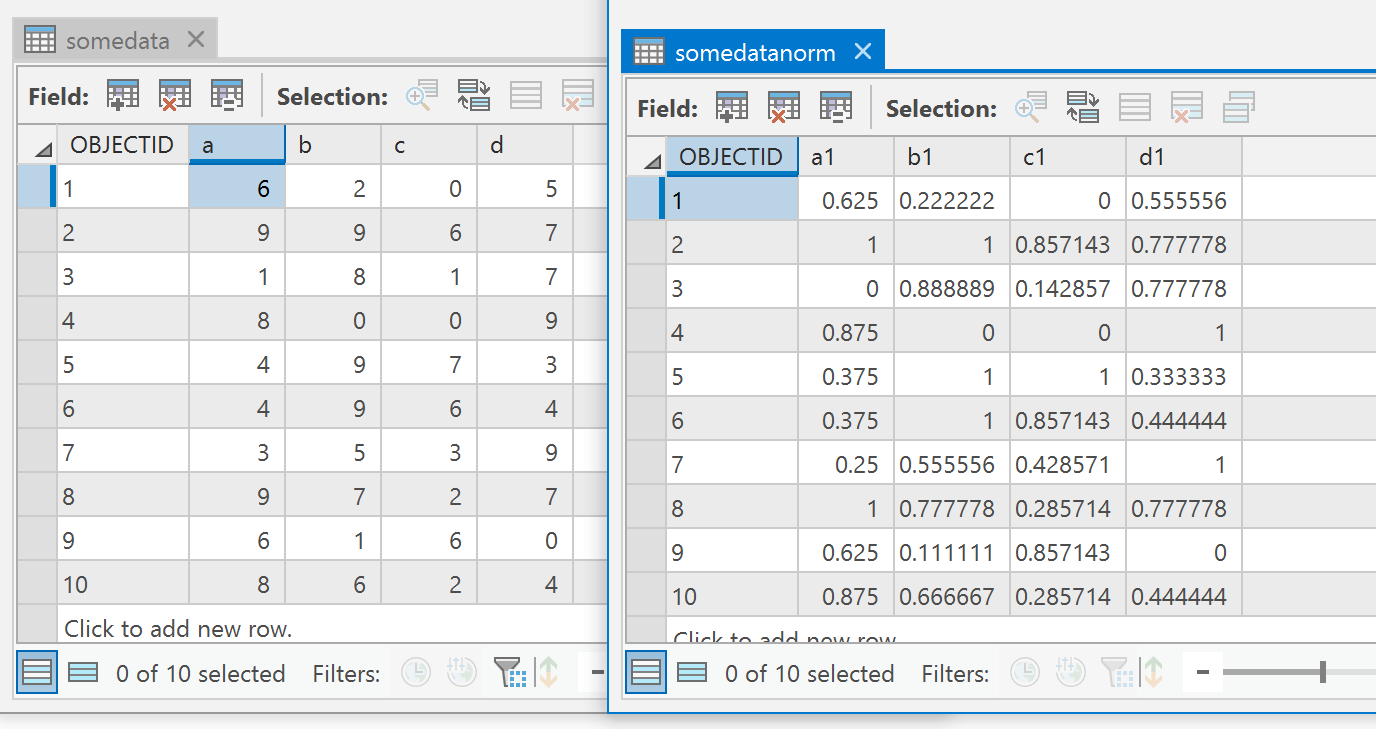# Math and Stats with NumPy... Normalize data

1572
3
03-18-2019 01:02 AM
Labels (1)byMVP Esteemed Contributor
2 3 1,572

Short one

Came up in a question.  I sadly suggested a spreadsheet.  To correct this, here is the numpy solution.

Normalizing data...

Here is the input and output tables``````names = ['a', 'b', 'c', 'd']
a = arcpy.da.TableToNumPyArray(out_tbl, names)
a0 = a.view('f8').reshape(a.shape, len(names))
dt = [('a1', 'f8'), ('b1', 'f8'), ('c1', 'f8'), ('d1', 'f8')]
n = normalize(a0)
new_names = ['a1', 'b1', 'c1', 'd1']
out = np.zeros((n.shape,), dtype=dt)
for i, name in enumerate(new_names):
out[name] = n[:, i]
arcpy.da.NumPyArrayToTable(out, out_tbl+"norm")‍‍‍‍‍‍‍‍‍‍‍‍‍‍‍‍‍‍‍‍‍‍‍‍‍‍‍‍‍‍‍‍‍‍‍‍‍‍‍‍``````
``````def normalize(a):
# a is a (n x dimension) np.array
tmp = a - np.min(a, axis=0)
out = tmp / np.ptp(tmp, axis=0)
return out‍‍‍‍‍‍‍‍‍‍‍‍‍‍‍‍‍‍‍‍‍‍‍‍‍``````

Line 1 and 2, read the table from ArcGIS Pro

Line 3, 'view' the array as a floating point numbers.

Line 4, create an output data type for sending it back

Line 5, normalize the data

Lines 6 to 10, bumpfh to send it back to Pro as a table

Normalize... hope I got it right... take the array, subtract the min then divide by the range.  np.ptp is the 'point-to-point' function which is the range

Normalize by row, column or overall

Now, lets assume that an input dataset could be data arranged by row, column or as a raster...  We need to change of normalize equation just a bit to see the results.

``````# ---- Adding an axis parameter ----

def normalize(a, axis=None):
# a is a (n x dimension) np.array
tmp = a - np.min(a, axis=axis)
out = tmp / np.ptp(tmp, axis=axis)
return out

a = np.arange(25).reshape(5,5)   # ---- some data

array([[ 0,  1,  2,  3,  4],
[ 5,  6,  7,  8,  9],
[10, 11, 12, 13, 14],
[15, 16, 17, 18, 19],
[20, 21, 22, 23, 24]])

normalize(a, axis=0)     # ---- normalize by column

array([[0.  , 0.  , 0.  , 0.  , 0.  ],
[0.25, 0.25, 0.25, 0.25, 0.25],
[0.5 , 0.5 , 0.5 , 0.5 , 0.5 ],
[0.75, 0.75, 0.75, 0.75, 0.75],
[1.  , 1.  , 1.  , 1.  , 1.  ]])

normalize(a, axis=1)     # ---- normalize by row

array([[ 0.  , -0.25, -0.5 , -0.75, -1.  ],
[ 0.31,  0.06, -0.19, -0.44, -0.69],
[ 0.62,  0.38,  0.12, -0.12, -0.38],
[ 0.94,  0.69,  0.44,  0.19, -0.06],
[ 1.25,  1.  ,  0.75,  0.5 ,  0.25]])

normalize(a, axis=None)  # ---- normalize overall

array([[0.  , 0.04, 0.08, 0.12, 0.17],
[0.21, 0.25, 0.29, 0.33, 0.38],
[0.42, 0.46, 0.5 , 0.54, 0.58],
[0.62, 0.67, 0.71, 0.75, 0.79],
[0.83, 0.88, 0.92, 0.96, 1.  ]])
‍‍‍‍‍‍‍‍‍‍‍‍‍‍‍‍‍‍‍‍‍‍‍‍‍‍‍‍‍‍‍‍‍‍‍‍‍‍‍‍``````

Lots of stuff you can do